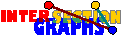# Examples

1) Students visit courses . Every student has a list of courses she or he visits, and every course has a list of attendant students. However, these lists are not available to the public. Now the courses have to be scheduled in such a way that no two courses with common students have overlapping meeting times. Somehow the administration samples the information which courses have common students. This information is visualized as the `conflict graph', where the courses are the vertices, and two such vertices are joined by an edge iff there is some time conflict, i.e. if the courses have common students.

2) Five persons enter some train at different doors in Santiago, and leave in Concepcion at different doors. During the journey, some of them move along the train. The train is narrow enough that people passing each other must see themselves. During the journey, A saw B and E, B saw A and C, C saw B and D, D saw C and E, and E saw D and A. Is that possible?

 3) The straight lines in Figure 1 are airplane routes. They have to be assigned different flight altitudes such that, for security reasons, intersecting lines get different altitudes. How many different altitudes are necessary?4) A new service at a movie theatre is to ask every viewer the range of brightness and the range of sound volume he or she would accept. According to these votes the projectionist chooses that adjustment that satisfies most people. How does he find it?

5) Consider the following 0-1 optimization problem. Given an nm 0-1 matrix A, the task is to find x{0,1}m obeying A x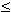1, and maximizing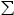i=1...m xi.

Example 2 is a recognition problem, i.e. the question whether or not some graph is the intersection graph of sets of a certain shape. In examples 3 and 4 we have an optimization problem over some family of sets. Cruical is that the parameter only depends on which of the sets intersects. Then we may and do attack the problem by viewing the intersection graph. The parameter which has to be optimized usually is a well-known graph parameter. For instance, in example 3 we are trying to `color' the vertices of the intersection graph by altitutes in such a way that adjacent vertices get different colors---this is just the well-known graph coloring problem. In Example 4 it turns out that we are interested in a maximum clique of the intersection graph, i.e. a maximum set of pairwise adjacent vertices. In Example 5 we can also reformulate the optimizatiuon problem into a graph optimization problem: Consider the columns of A as subsets of {0,1, ... , m} (according to the 0-1 pattern). Then the 0-1 vectors x obeying Ax1 are just the independent sets of the intersection graph of the columns of A, and the optimization problem can be reformulated to finding a maximum independent set in this graph.

So what is the advantage of the reformulation as a graph problem? Firstly we delete unnecessary information and may concentrate on the essential. Moreover, even though the problems may and will be NP-complete in general, there is a huge amount of knowledge about such graph optimization problems, and one could try heuristics, approximation algorithms, and so on.

Example 6: Let k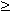2 be any integer. Assume that every student in Example 1 visits exactly k courses. Assume students know each other if and only if they attend some common course. All students are asked who knows whom. Is it possible to reconstruct all attendance lists?

 7) We modify the overeater example by assuming that we no longer have mathematicians but members of a dancing club which only remember people of the other sex. Each member claims that she or he had a connected attendance time. Is the data to the right compatible with the model? Who, if any, is lying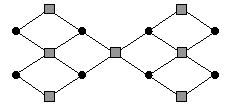8) For some animals we draw an "A-eats-B" digraph. The competition graph of that digraph has the animals as vertices, and distinct animals are connected if something is eaten by both of them. In the resource graph, two distinct animals are connected by an edge if something eats both of them. Now assume the competition graph is an interval graph. What can be said about the resource graph?

...

Back to the start page for intersection graphs.

Erich Prisner